Tamilnadu State Board New Syllabus Samacheer Kalvi 8th Maths Guide Pdf Chapter 1 Numbers Ex 1.6 Textbook Questions and Answers, Notes.

## Tamilnadu Samacheer Kalvi 8th Maths Solutions Chapter 1 Numbers Ex 1.6

Question 1.
Fill in the blanks.
(i) (-1)even integer is __________ .
1

(ii) For a ≠ 0, a0 is __________ .
1(iii) 4-3 × 5-3 = __________ .
20-3

(iv) (-2)-7 is = __________ .
$$\frac{-1}{128}$$

(v) $$\left(-\frac{1}{3}\right)^{-5}$$ = _________ .
– 243

Question 2.
Say True or False:
(i) If 8x = $$\frac { 1 }{ 64 }$$, the value of x is -2.
True

(ii) The simplified form of $$(256)^{\frac{-1}{4}} \times 4^{2}$$ is $$\frac{1}{4}$$.
True(iii) Using the power rule, $$\left(3^{7}\right)^{-2}$$ = 35
True

(iv) The standard form of 2 × 10-4 is 0.0002.
False

(v) The scientific form of 123.456 is 1.23456 × 10-2.
True

Question 3.
Evaluate
(i) $$\left(\frac{1}{2}\right)^{3}$$
(ii) $$\left(\frac{1}{2}\right)^{-5}$$
(iii) $$\left(\frac{-5}{6}\right)^{-3}$$
(iv) (2-5 × 27) ÷ 2-2
(v) (2-1 × 3-1) ÷ 6-2
(i) $$\left(\frac{1}{2}\right)^{3}$$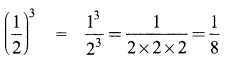(ii) $$\left(\frac{1}{2}\right)^{-5}$$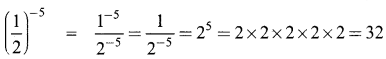(iii) $$\left(\frac{-5}{6}\right)^{-3}$$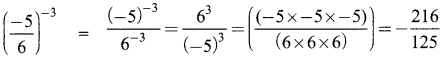(iv) (2-5 × 27) ÷ 2-2
(2-5 × 27) ÷ 2-2 = (2-5 + 7) ÷ 2-2
= 22 ÷ 2-2
= 22+2
= 24
= 16

(v) (2-1 × 3-1) ÷ 6-2
(2-1 × 3-1) ÷ 6-2 = (2 × 3)-1 ÷ 6-2
= (6-1) ÷ 6-2
= 6(-1)-(-2)
= 61
= 6Question 4.
Evaluate
(i) $$\left(\frac{2}{5}\right)^{4} \times\left(\frac{5}{2}\right)^{-2}$$
(ii) $$\left(\frac{4}{5}\right)^{-2} \div\left(\frac{4}{5}\right)^{-3}$$
(iii) $$2^{7} \times\left(\frac{1}{2}\right)^{-3}$$
(i) $$\left(\frac{2}{5}\right)^{4} \times\left(\frac{5}{2}\right)^{-2}$$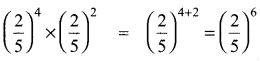(ii) $$\left(\frac{4}{5}\right)^{-2} \div\left(\frac{4}{5}\right)^{-3}$$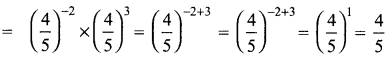(iii) $$2^{7} \times\left(\frac{1}{2}\right)^{-3}$$
= 27 × 23
= 27 + 3
= 210

Question 5.
Evaluate:
(i) (50 + 6-1) × 32
(ii) (2-1 + 3-1) ÷ 6-1
(iii) (3-1 + 4-2 + 5-3)0
(i) (50 + 6-1) × 32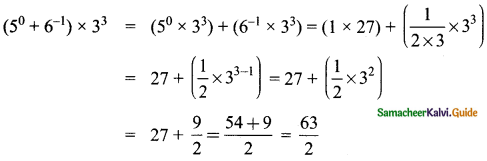(ii) (2-1 + 3-1) ÷ 6-1
(2-1 + 3-1) ÷ 6-1 = $$\left(\frac{1}{2}+\frac{1}{3}\right)$$ + 6-1
= $$\left(\frac{3+2}{6}\right)$$ + 6-1 = $$\left(\frac{5}{6}\right)$$ + 6-1 = $$\frac{5}{6}$$ × 6 = 5

(iii) (3-1 + 4-2 + 5-3)0
(3-1 + 4-2 + 5-3)0 = 1
[∵ a0 = 1 where a ≠ 0]

Question 6.
Simplify
(i) (32)3 × (2 × 35)-2 × (18)2
(ii) $$\frac{9^{2} \times 7^{3} \times 2^{5}}{84^{3}}$$
(iii) $$\frac{2^{8} \times 2187}{3^{5} \times 3^{2}}$$
(i) (32)3 × (2 × 35)-2 × (18)2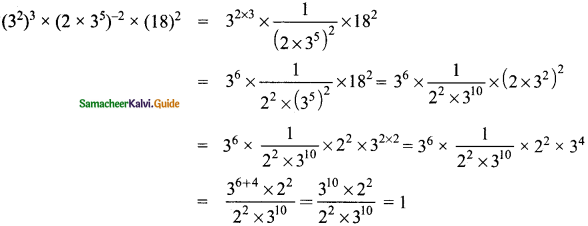(ii) $$\frac{9^{2} \times 7^{3} \times 2^{5}}{84^{3}}$$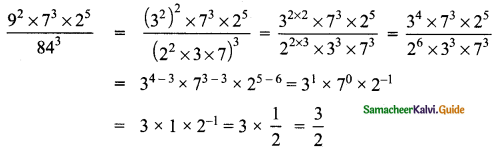(iii) $$\frac{2^{8} \times 2187}{3^{5} \times 3^{2}}$$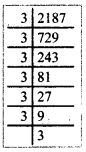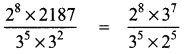= 28-5 × 37-5
= 23 × 32
= 8 × 9
= 72Question 7.
Solve for x:
(i) $$\frac{2^{2 x-1}}{2^{x+2}}$$ = 4
(ii) $$\frac{5^{5} \times 5^{-4} \times 5^{x}}{5^{12}}$$ = 5-5
(i) $$\frac{2^{2 x-1}}{2^{x+2}}$$ = 4
22x – 1 – (x + 2) = 22
22x – 1 – x – 2) = 22
22x – 3 = 22
Equating the powers of the same base 2.
x – 3 = 2
x – 3 + 3 = 2 + 3
x = 5

(ii) $$\frac{5^{5} \times 5^{-4} \times 5^{x}}{5^{12}}$$ = 5-5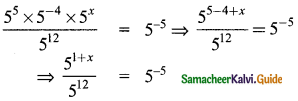⇒ 51 + x – 12 = 5-5
⇒ 5x – 11 = 5-5
Equating the powers of same base 5.
x – 11 = – 5
x – 11 + 11 = – 5 + 11
x = 6Question 8.
Expand using exponents:
(i) 6054.321
(ii) 897.14
(i) 6054.321
6054.321 = (6 × 1000) + (0 × 100) + (5 × 10) + (4 × 100) + $$\frac{3}{10}+\frac{2}{100}+\frac{1}{1000}$$
= (6 × 103) + (5 × 101) + (4 × 100) + $$\frac{3}{10}+\frac{2}{100}+\frac{1}{1000}$$
= (6 × 103) + (5 × 101) + (4 × 100) + (3 × 10-1) + (2 × 10-2) + (1 × 10-3)

(ii) 897.14
= (8 × 100) + (9 × 10) + (7 × 100) + $$\frac{1}{10}+\frac{4}{100}$$
= (8 × 1o2) +( 9 × 101) + (7 × 100) + $$\left(1 \times \frac{1}{10}\right)+\left(4 \times \frac{1}{100}\right)$$
= (8 × 103) + (9 × 103) + (7 × 100) + (1 × 10-1) + (4 × 10-2)

Question 9.
Find the number is standard form:
(i) 8 × 104 + 7 × 103 + 6 × 102 + 5 × 101 + 2 × 1 + 4 × 10-2 + 7 × 10-4
(ii) 5 × 103 + 5 × 101 + 5 × 10-1 + 5 × 10-3
(iii) The radius of a hydrogen atom is 2.5 × 10-11 m
(i) 8 × 104 + 7 × 103 + 6 × 102 + 5 × 101 + 2 × 1 + 4 × 10-2 + 7 × 10-4
= 8 × 104 + 7 × 103 + 6 × 102 + 5 × 101 + 2 × 1 + 4 × 10-2 + 7 × 10-4
= 8 × 10000 + 7 × 1000 + 6 × 100 + 5 × 10 + 2 × 1 + 4 × $$\frac{1}{100}$$ + 7 × $$\frac{1}{10000}$$
= 80000 + 7000 + 600 + 50 + 2 + $$\frac{4}{100}$$ + $$\frac{7}{10000}$$
= 87652.0407(ii) 5 × 103 + 5 × 101 + 5 × 10-1 + 5 × 10-3
= 5 × 103 + 5 × 101 + 5 × 10-1 + 5 × 10-3
= 5 × 1000 + 5 × 10 + 5 × $$\frac{1}{10}$$ + 5 × $$\frac{1}{1000}$$
= 5000 + 50 + $$\frac{5}{10}+\frac{5}{1000}$$ = 5050.505

(iii) The radius of a hydrogen atom is 2.5 10-11 m
Radiys of a hydrogen atom = 2.5 × 10-11 m
= $$2.5 \times \frac{1}{10^{11}} \mathrm{m}=\frac{2.5}{10^{11}} \mathrm{m}$$
= 0.000000000025 m

Question 10.
Write the following numbers in scientific notation:
(i) 467800000000
467800000000 = 4.678 × 1011

(ii) 0.000001972
0.000001972 = 1.972 × 10-6

(iii) 1642.398
1642.398 = 1.642398 × 103(iv) Earth’s volume is about 1,083,000,000,000 cubic kilometres
1,083,000,000,000
Earth’s volume = 1.083 110 × 102 cubic kilometres

(v) If you fill a bucket with dirt, the portion of the whole Earth that is in the bucket will be 0.00000000000000000000000 16 kg
Portion of earth in the bucket = 0.00000000000000000000000 16 kg
= 1.6 10 × 1024 kg.

Objective Type Questions

Question 11.
By what number should (-4)-1 be multiplied so that the product becomes 10-1?
(A) $$\frac{2}{3}$$
(B) $$\frac{-2}{5}$$
(C) $$\frac{5}{2}$$
(D) $$\frac{-5}{2}$$
(B) $$\frac{-2}{5}$$
Hint:
(-4)-1 = $$\left(-\frac{1}{4}\right)^{1}=\frac{-1}{4}$$Question 12.
(-2)-3 × (-2)-2 = ___________.
(A) $$\frac{-1}{32}$$
(B) $$\frac{1}{32}$$
(C) 32
(D) -32
(A) $$\frac{-1}{32}$$

Question 13.
Which is not correct?
(A) $$\left(\frac{-1}{4}\right)^{2}$$ = 4-2
(B) $$\left(\frac{-1}{4}\right)^{2}=\left(\frac{1}{2}\right)^{4}$$
(C) $$\left(\frac{-1}{4}\right)^{2}$$ = 16-1
(D) $$-\left(\frac{1}{4}\right)^{2}$$ = 16-1
$$-\left(\frac{1}{4}\right)^{2}$$ = 16-1
Hint:
(-2) – 3 x (- 2) – 2 = (-2) – 3 – 2 = (-2) – 5 ($$-\frac { 1 }{ 2 }$$)5 = $$-\frac { 1 }{ 32 }$$

Question 14.
If $$\frac{10^{x}}{10^{-3}}$$ = 109, then x is ___________ .
(A) 4
(B) 5
(C) 6
(D) 7Question 15.
0.0000000002020 in scientific form is __________ .
(A) 2.02 × 109
(B) 2.02 × 10-9
(C) 2.02 × 10-8
(D) 2.02 × 10-10